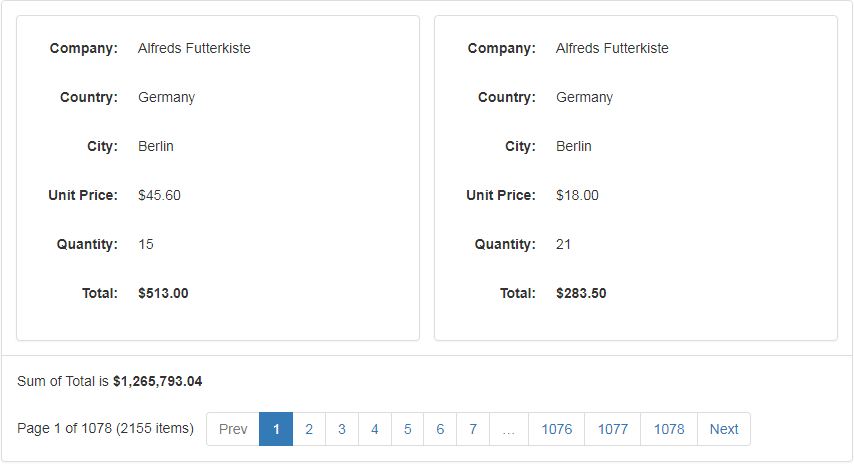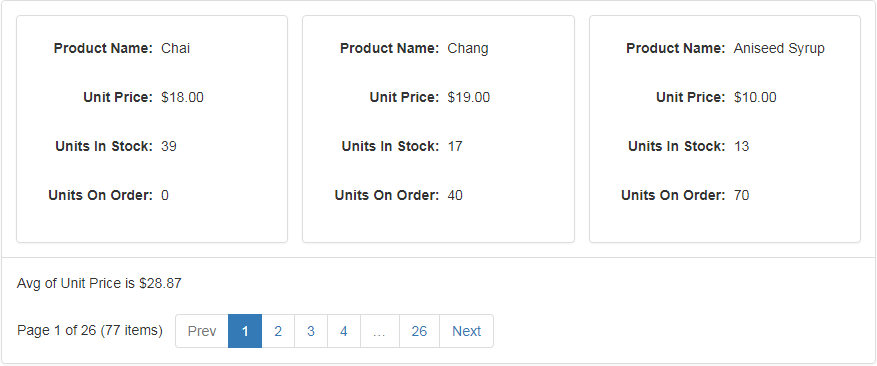21.2 (EAP/Beta)
21.1
20.2
20.1
19.2
19.1
18.2
18.1
17.2

# Data Summaries

The Bootstrap Card View control allows you to display a summary, i.e., brief information about data columns. For example, you can display the number of records or the maximum value.

The Bootstrap Card View supports total summaries that are calculated over all the cards and are displayed within the Summary Panel.Summaries are represented by ASPxSummaryItem objects. To work properly, the following two summary item properties must be specified.

``````<dx:ASPxSummaryItem FieldName="Total" SummaryType="Sum"/>
``````

The summary item above summarizes the ‘Total’ field values within cards and displays the sum in the summary panel.

Note

In server mode, a summary cannot be calculated for unbound columns in which values are calculated using events (see BootstrapCardView.CustomUnboundColumnData). Only columns with unbound expressions (see CardViewColumn.UnboundExpression) support summary calculation.

## Example: Creating Total Summaries in Code

This example shows how to calculate the average price and display it in the Card View’s summary panel:``````ASPxCardViewSummaryItem totalSummary = new ASPxCardViewSummaryItem();
totalSummary.FieldName = "UnitPrice";
totalSummary.SummaryType = DevExpress.Data.SummaryItemType.Average;